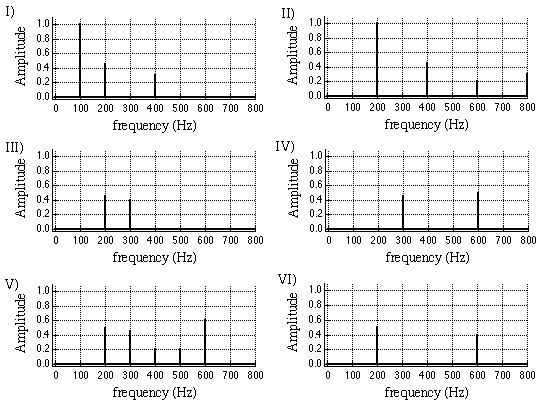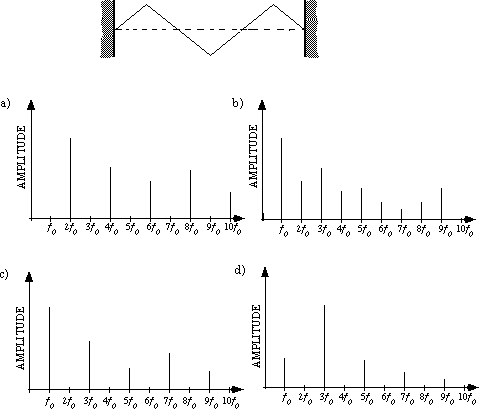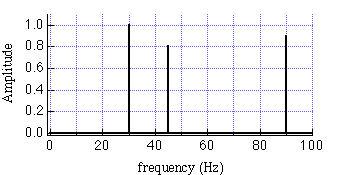TUTORIAL QUESTIONS 240-270

240.  The frequency spectra of six complex waves are shown below.  Which waves would you perceive to have the same pitch as I) if they were played over a loud speaker?a) III and V would have the same pitch as I.
b) All would have the same pitch as I.
c) Only V has the same pitch as I.
d) Only IV has the same pitch as I.
e) None of the sounds would have the same pitch as I.

Fundamentals of Sound reference:Sec. 7-D.

250.Which frequency spectrum below most likely corresponds to the following standing complex wave on a string tied at both ends?abcd)

Fundamentals of Sound reference:Secs. 7-B.

255.  What is the repeat time of a complex wave made up by adding together (by superposition) the three sinusoidal waves having frequencies 18 Hz, 24 Hz, and 60 Hz?

a)  6 seconds
b) 18 seconds
c) 34 seconds
d) 1/34 second
e) 1/6 second
f) 1/18 second

Fundamentals of Sound reference:Secs. 7-D.

260.   The repeat time of any complex wave on a string tied at both ends is always equal to, or less than the period of the fundamental.

a) true
b) false

Fundamentals of Sound reference:Secs. 7-D.

270.  Below is a frequency spectrum of a complex standing wave on a string tied at both ends.  The allowed normal modes on the string form the harmonic series, {5 Hz, 10 Hz, 15 Hz, ...}.What is the repeat frequency of this complex wave?

Fundamentals of Sound reference:Sec. 7-D.